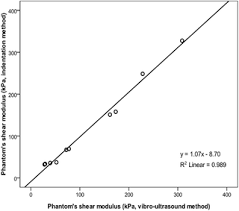How to Calculate and Solve for Shear Modulus | Rock MechanicsThe image above represents shear modulus.

To compute for shear modulus, two essential parameters are needed and these parameters are young’s modulus (E) and Poisson’s ratio (v).

The formula for calculating the shear modulus:

G = E / 2(1 + v)

Where:

G = Shear Modulus
E = Young’s Modulus
v = Poisson’s Ratio

Let’s solve an example;
Find the shear modulus when the young’s modulus is 32 and the Poisson’s ratio is 24.

This implies that;

E = Young’s Modulus = 32
v = Poisson’s Ratio = 24

G = E / 2(1 + v)
G = 32 / 2(1 + 24)
G = 32 / 2(25)
G = 32 / 50
G = 0.64

Therefore, the shear modulus is 0.64.

Calculating the Young’s Modulus when the Shear Modulus and the Poisson’s Ratio is Given.

E = G (2 + 2v)

Where:

E = Young’s Modulus
G = Shear Modulus
v = Poisson’s Ratio

Let’s solve an example;
Find the young’s modulus when the shear modulus is 12 and the Poisson’s ratio is 10.

This implies that;

G = Shear Modulus = 12
v = Poisson’s Ratio = 10

E = G (2 +2v)
E = 12 (2 + 2(10))
E = 12 (2 + 20)
E = 12 (22)
E = 264

Therefore, the young’s modulus is 264.

Calculating the Poisson’s Ratio when the Shear Modulus and the Young’s Modulus is Given.

v = E – 2G / 2G

Where:

v = Poisson’s Ratio
G = Shear Modulus
E = Young’s Modulus

Let’s solve an example;
Find the Poisson’s ratio when the young’s modulus is 48 and the shear modulus is 18.

This implies that;

G = Shear Modulus = 18
E = Young’s Modulus = 48

v = E – 2G / 2G
v = 48 – 2(18) / 2 x 18
v = 48 – 36 / 36
v = 12 / 36
v = 0.33

Therefore, the Poisson’s ratio is 0.33.

Nickzom Calculator – The Calculator Encyclopedia is capable of calculating the shear modulus.

To get the answer and workings of the shear modulus using the Nickzom Calculator – The Calculator Encyclopedia. First, you need to obtain the app.

You can get this app via any of these means:

To get access to the professional version via web, you need to register and subscribe for NGN 1,500 per annum to have utter access to all functionalities.
You can also try the demo version via https://www.nickzom.org/calculator

Android (Paid) – https://play.google.com/store/apps/details?id=org.nickzom.nickzomcalculator
Android (Free) – https://play.google.com/store/apps/details?id=com.nickzom.nickzomcalculator
Apple (Paid) – https://itunes.apple.com/us/app/nickzom-calculator/id1331162702?mt=8
Once, you have obtained the calculator encyclopedia app, proceed to the Calculator Map, then click on Geology under Add-on.Now, Click on Rock Mechanics under GeologyNow, Click on Shear Modulus under Rock MechanicsThe screenshot below displays the page or activity to enter your values, to get the answer for the shear modulus according to the respective parameters which are the young’s modulus (E) and Poisson’s ratio (v).Now, enter the values appropriately and accordingly for the parameters as required by the young’s modulus (E) is 32 and Poisson’s ratio (v) is 24.Finally, Click on CalculateAs you can see from the screenshot above, Nickzom Calculator– The Calculator Encyclopedia solves for the shear modulus and presents the formula, workings and steps too.